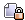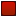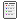###Author Topic: Bulldozed And Scorched Tile Indexing  (Read 841 times)

####Hooman

• Hero Member
••• Posts: 4832##### Bulldozed And Scorched Tile Indexing
« on: July 06, 2008, 12:19:19 AM »
I noticed a slight oddity in some code where tile index 10 is always considered to be a bulldozed tile index. This is hardcoded into a routine to check if a tile index is a bulldozed tile index, which is never called anywhere that I can see, but it does seem to be inlined in a function that is called. Also, when bulldozing a tile, if there is no mapping from the source tile to a bulldozed tile, then it will use tile index 10 for the bulldozed tile index.

Scorched/burned tiles have a different mapping depending on if the terrain is bulldozed or not. Hence the 10 above is relevant to this. There are 3 tiles for each of the normal and bulldozed terrain. It randomly chooses one.

Here's an attempt to reverse the code into something C++ like. I chose to use "continue" instead of nested if statements, since I didn't have to complement that branch condition that way. I tend to make mistakes when I complement branch conditions.

Code: [Select]
``// Note: tileIndex 10 is always considered to be bulldozedIsBulldozedTileIndex(int tileIndex){	int i;	for (i = 0; i < numTerrainTypes; i++)	{  if (terrainType[i]->bulldozedTileIndex > tileIndex) continue;  if (terrainType[i]->bulldozedTileIndex + 1 > tileIndex) return true;	// (bulldozedTileIndex == tileIndex)	}	return (tileIndex == 10);}IsScorchedTileIndex(int tileIndex){	int i;	for (i = 0; i < numTerrainTypes; i++)	{  scorchedTileIndex = terrainType[i].scorchedTileIndex;  if (scorchedTileIndex == 0) continue;  if (tileIndex < scorchedTileIndex) continue;  if (scorchedTileIndex + 6 > tileIndex) return true;	// (scorchedTileIndex <= tileIndex < scorchedTileIndex + 6)	}	return false;}GetScorchMarkTileIndex(int tileIndex){	int i, j;	int newTileIndex = 0;	for (i = 0; i < numTerrainTypes; i++)	{  if (terrainType[i]->firstTile > tileIndex) continue;  if (terrainType[i]->lastTile < tileIndex) continue;  newTileIndex = terrainType[i]->scorchedTileIndex;  if (newTileIndex == 0) continue;  for (j = 0; j < numTerrainTypes; j++)  {  	if (terrainType[j]->bulldozedTileIndex > tileIndex) continue;  	if (terrainType[j]->bulldozedTileIndex + 1 > tileIndex) continue(2);	// (bulldozedTileIndex == tileIndex)  }  if (tileIndex == 10) continue;  // Increment to non bulldozed scorched tile index  newTileIndex += 3;  	// **  ((tileIndex != 10) && (tileIndex != bulldozedTileIndex[0..?]))	}	if (newTileIndex != 0)	{  newTileIndex += Rand(3);	}	return newTileIndex;}``

Note that there are 9 burn tiles for the rock tile set, but this code only ever makes use of 6 tiles per terrain type. (Granted, you can have more than 1 terrain type object for a tile set). There is also more code to check if a tile index is a scorched/burn tile index, and it checks for a range of 6 tiles from the base scorch/burn index.